COS 126 Recursive Graphics Programming Assignment

Write a program that plots a Sierpinski triangle, as illustrated below. Then develop a program that plots a recursive patterns of your own design.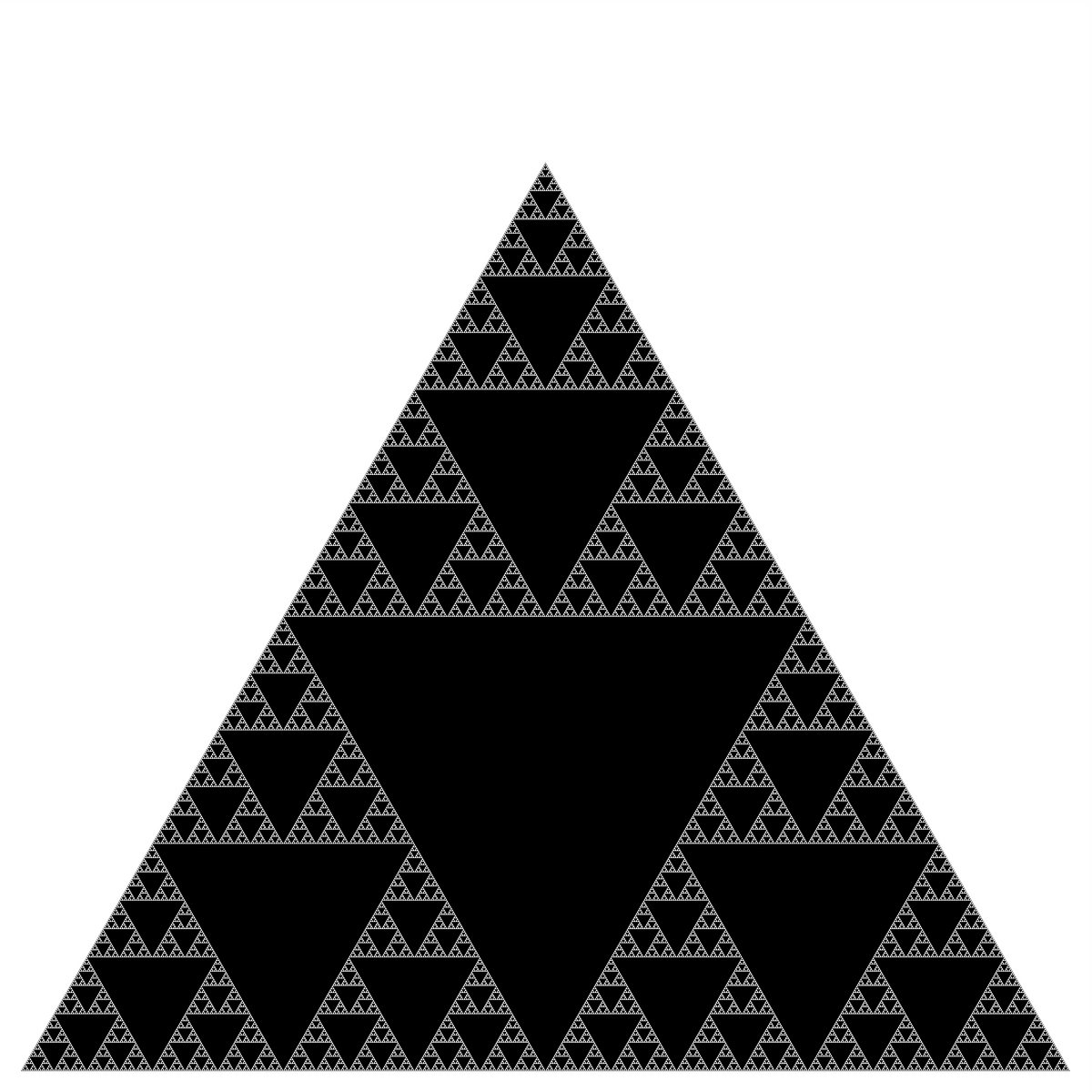Part 1.   The Sierpinski triangle is another example of a fractal pattern like the H-tree from Section 2.3. The pattern was described by Polish mathematician Waclaw Sierpinski in 1915, but has appeared in Italian art since the 13th century. Though the Sierpinski triangle looks complex, it can be generated with a short recursive program. Your task is to write a program Sierpinski.java with a recursive function sierpinski() and a main() function that calls the recursive function once, and plots the result using standard drawing. Think recursively: your function should draw one black triangle (pointed downwards) and then call itself recursively 3 times (with an appropriate stopping condition). When writing your program, exercise modular design: include a (non-recursive) function triangle() that draws an equilateral triangle of a specified size at a specified location.

Your program shall take one integer command-line argument N, to control the depth of the recursion. First, make sure that your program draws a single black triangle when N is set to 1. Then, check that it draws four black triangles when N is set to 2. Your program will be nearly (or completely) debugged when you get to this point. Below are the target Sierpinski triangles for different values of N.

 % java Sierpinski 1 % java Sierpinski 2 % java Sierpinski 3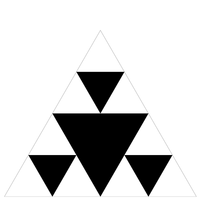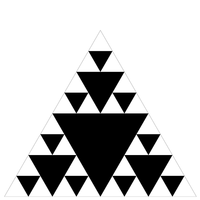% java Sierpinski 4 % java Sierpinski 5 % java Sierpinski 6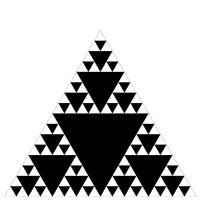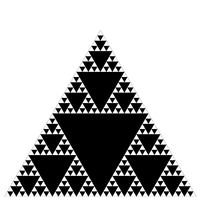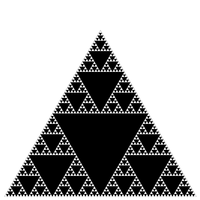A diversion: fractal dimension.   In grade school, we learn that the dimension of a line segment is one, the dimension of a square is two, and the dimension of a cube is three. But you probably didn't learn what is really meant by dimension. How can we express what it means mathematically or computationally? Formally, we can define the Hausdorff dimension or similarity dimension of a self-similar figure by partitioning the figure into a number of self-similar pieces of smaller size. We define the dimension to be the log (# self similar pieces) / log (scaling factor in each spatial direction). For example, we can decompose the unit square into 4 smaller squares, each of side length 1/2; or we can decompose it into 25 squares, each of side length 1/5. Here, the number of self-similar pieces is 4 (or 25) and the scaling factor is 2 (or 5). Thus, the dimension of a square is 2 since log (4) / log(2) = log (25) / log (5) = 2. We can decompose the unit cube into 8 cubes, each of side length 1/2; or we can decompose it into 125 cubes, each of side length 1/5. Therefore, the dimension of a cube is log(8) / log (2) = log(125) / log(5) = 3.

We can also apply this definition directly to the (set of white points in) Sierpinski triangle. We can decompose the unit Sierpinski triangle into 3 Sierpinski triangles, each of side length 1/2. Thus, the dimension of a Sierpinski triangle is log (3) / log (2) ≈ 1.585. Its dimension is fractional - more than a line segment, but less than a square! With Euclidean geometry, the dimension is always an integer; with fractal geometry, it can be any fraction. Fractals are widely applicable for describing physical objects like the coastline of Britain.

Part 2.   In this part you will design and create your own recursive fractal. Your job is to write a program Art.java that takes one integer command-line argument N to control the depth of recursion and produces a recursive pattern of your own choosing. It should work and stay within the drawing window for values 1 through 7. You are free to choose a geometric pattern (like, but not too similar to, Htree or Sierpinski) or a random construction (like, but not too similar to, Brownian). Originality and creativity in the design will be a factor in your grade.

Submission.   Submit Sierpinski.java, Art.java, readme.txt, and any supplementary image files needed by Art.java.